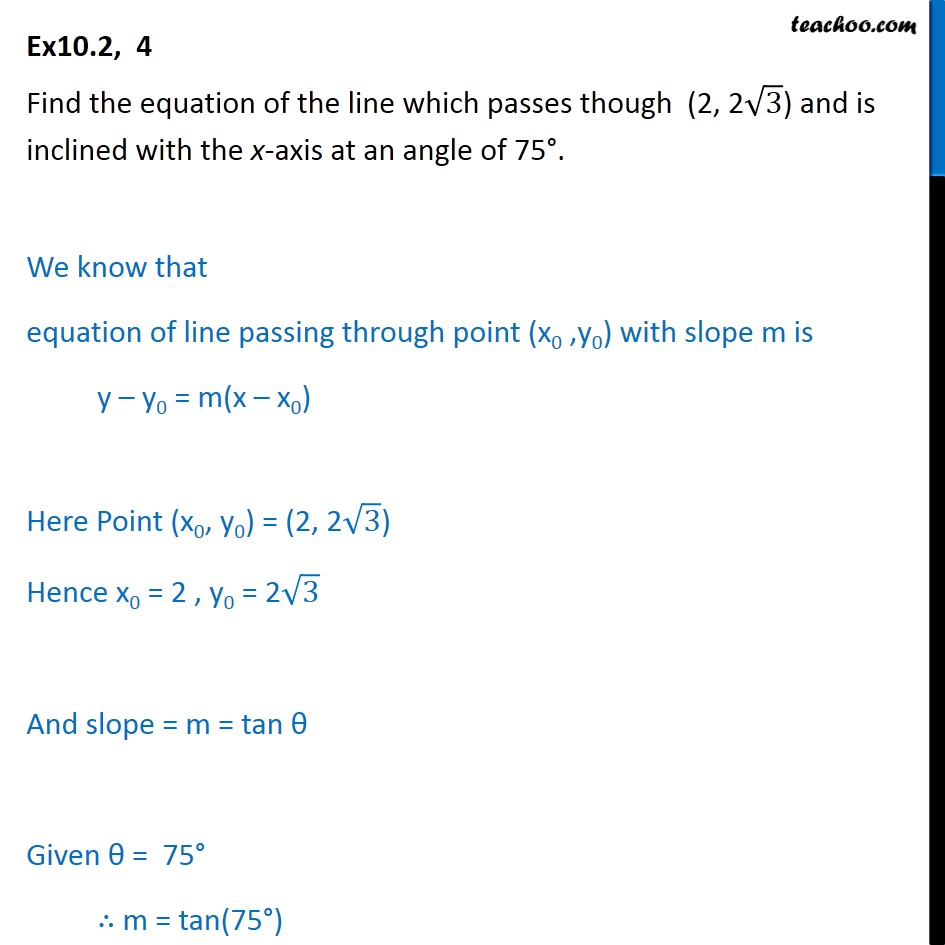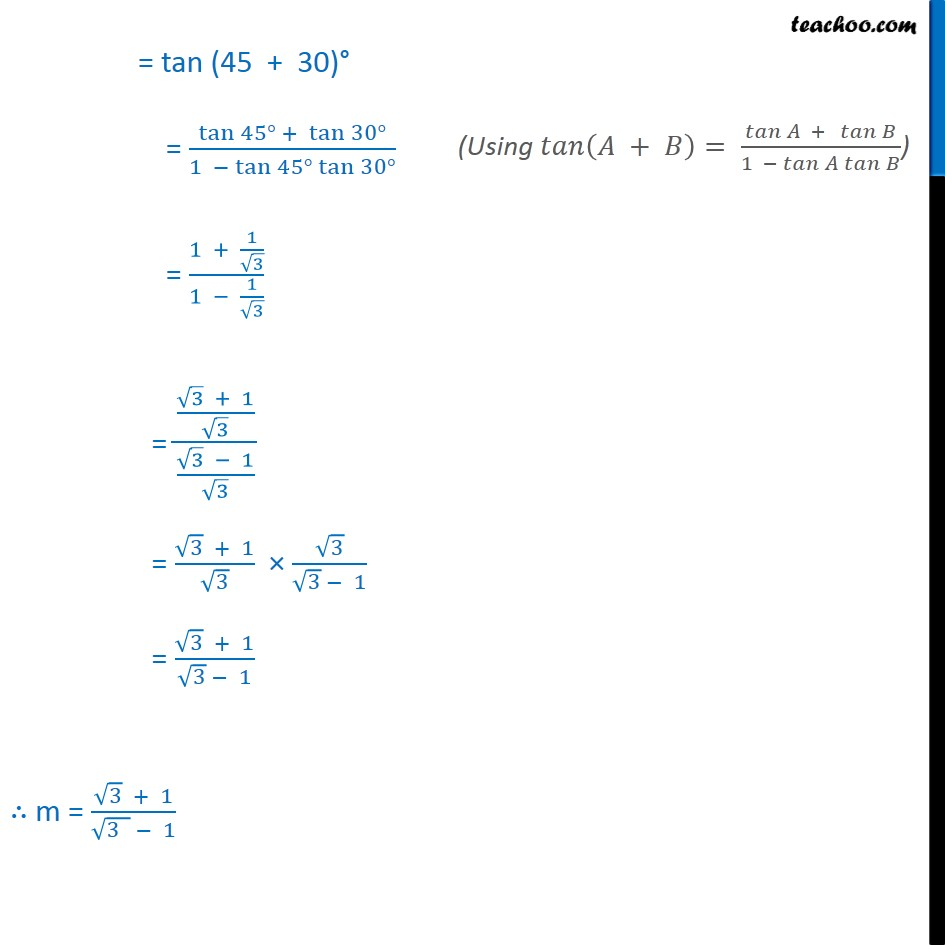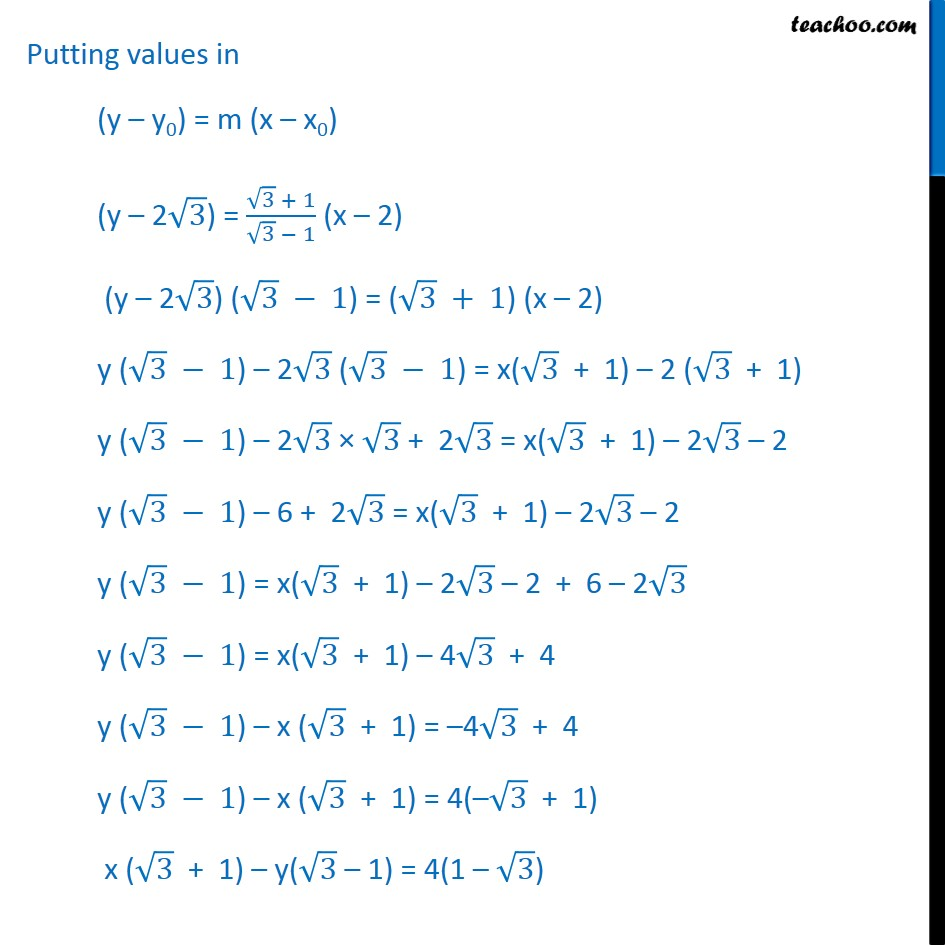1. Chapter 10 Class 11 Straight Lines
2. Serial order wise
3. Ex 10.2

Transcript

Ex10.2, 4 Find the equation of the line which passes though (2, 2 3) and is inclined with the x-axis at an angle of 75 . We know that equation of line passing through point (x0 ,y0) with slope m is y y0 = m(x x0) Here Point (x0, y0) = (2, 2 3) Hence x0 = 2 , y0 = 2 3 And slope = m = tan Given = 75 m = tan(75 ) = tan (45 + 30) = tan 45 + tan 30 /(1 tan 45 tan 30 ) = (1 + 1/ 3)/(1 1/ 3) = (( 3 + 1)/ 3)/(( 3 1)/ 3) = ( 3 + 1)/ 3 3/( 3 1) = ( 3 + 1)/( 3 1) m = ( 3 + 1)/( (3 ) 1) Putting values in (y y0) = m (x x0) (y 2 3) = ( 3 + 1)/( 3 1) (x 2) (y 2 3) ( 3 1) = ( 3 + 1) (x 2) y ( 3 1) 2 3 ( 3 1) = x( 3 + 1) 2 ( 3 + 1) y ( 3 1) 2 3 3 + 2 3 = x( 3 + 1) 2 3 2 y ( 3 1) 6 + 2 3 = x( 3 + 1) 2 3 2 y ( 3 1) = x( 3 + 1) 2 3 2 + 6 2 3 y ( 3 1) = x( 3 + 1) 4 3 + 4 y ( 3 1) x ( 3 + 1) = 4 3 + 4 y ( 3 1) x ( 3 + 1) = 4( 3 + 1) x ( 3 + 1) y( 3 1) = 4(1 3)

Ex 10.2